Select Page

# Interest Expense in a Monthly Financial Model (Cash Interest vs. Interest Expense)

• Peter Lynch

Interest expense is a period expense, so it appears in each period on your income statement in a financial model. Per some credit agreements, however, interest is only paid on a quarterly basis. Consequently, in a monthly financial model you will have periods with interest expense on the income statement without a corresponding cash outflow for interest paid (or cash interest). Template available for download at the bottom of this post.

To reconcile this timing difference, add a line item titled “Accrued Interest” to you balance sheet under current liabilities. This will pull from the supporting debt schedule, which will be amended as follows. First, add three line items just below the formula for Ending Principal Balance:

1. Interest Expense
2. Interest Accrued
3. Interest Paid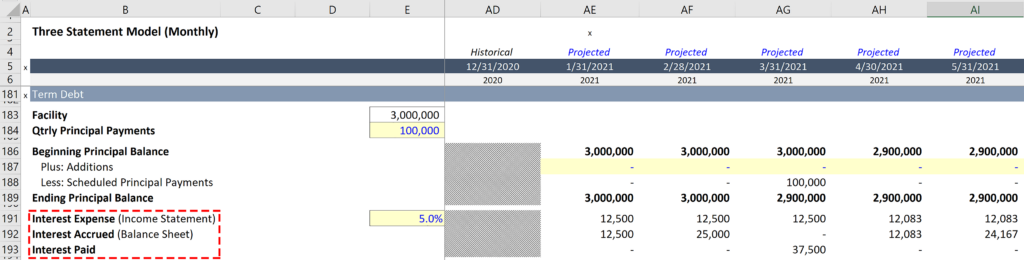Next, calculate interest expense for each period (see image). This will be similar to the approach used to project interest expense in a model with annual periods with two exceptions:

1. There is no need to take an average of the principal balance.
2. The interest rate needs to be adjusted to reflect the period of time.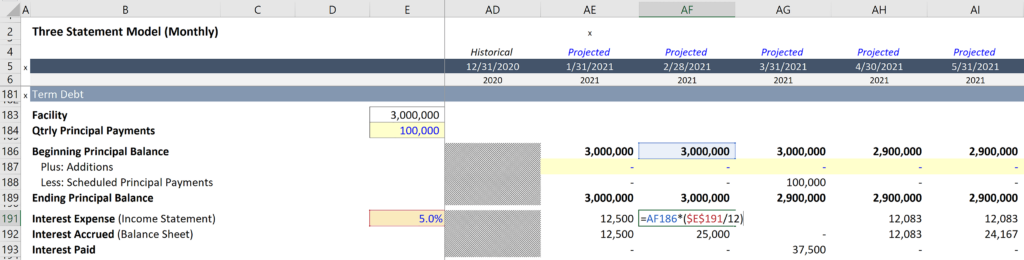Then calculate interest accrued on the line that follows. This is the line item that will link to the balance sheet to project accrued interest. To complete this calculation sum interest expense in the current period with interest accrued in the previous period, and then subtract interest paid in the current period (see image).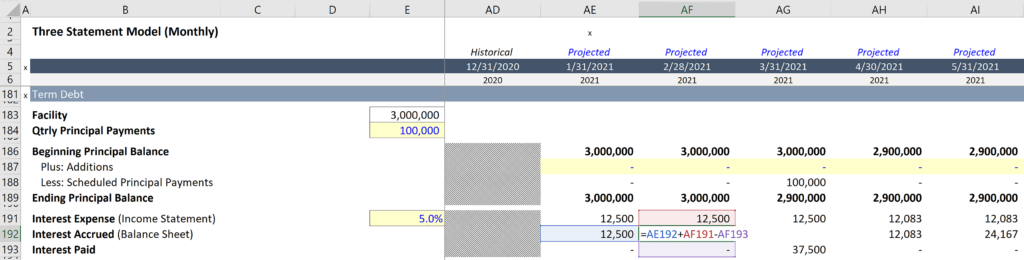Finally, in the last step related to the debt supporting schedule, we will calculate interest paid using the =IF() and =MOD() functions. The =MOD() function is a great way to confirm if the month is divisible by three, which makes it easy to identify a quarter (=MOD() video explanation). When the formula identifies a quarter end period it will return the sum of the previous three months of interest expense (see image).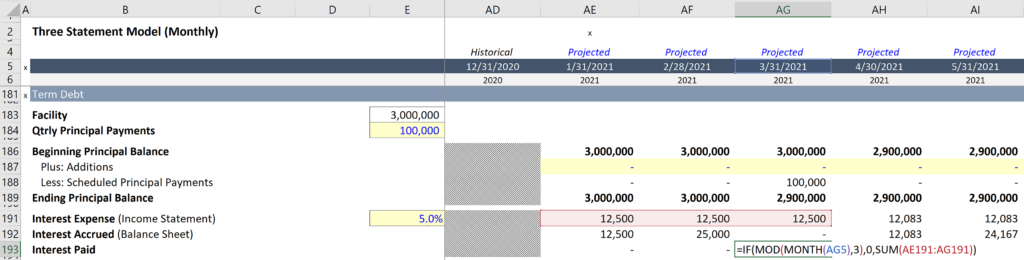Interest expense will link to the income statement in precisely the same way it does in an annual model, and now the Interest Accrued line item can link to Accrued Interest on the Balance sheet.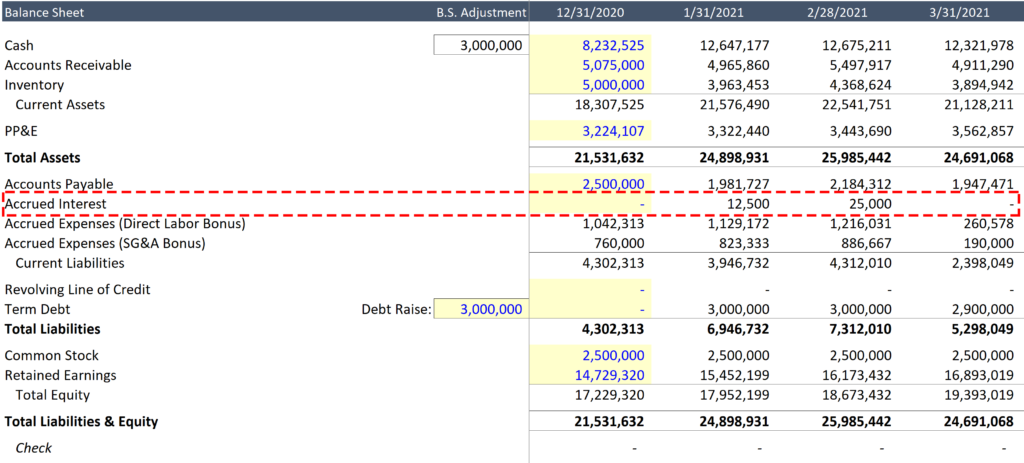The final step is to add a line item to the cash flow statement under changes in working capital. Title it “Accrued Interest” and subtract the current period from the prior period to reflect a cash outflow when the current balance declines from one period to the next.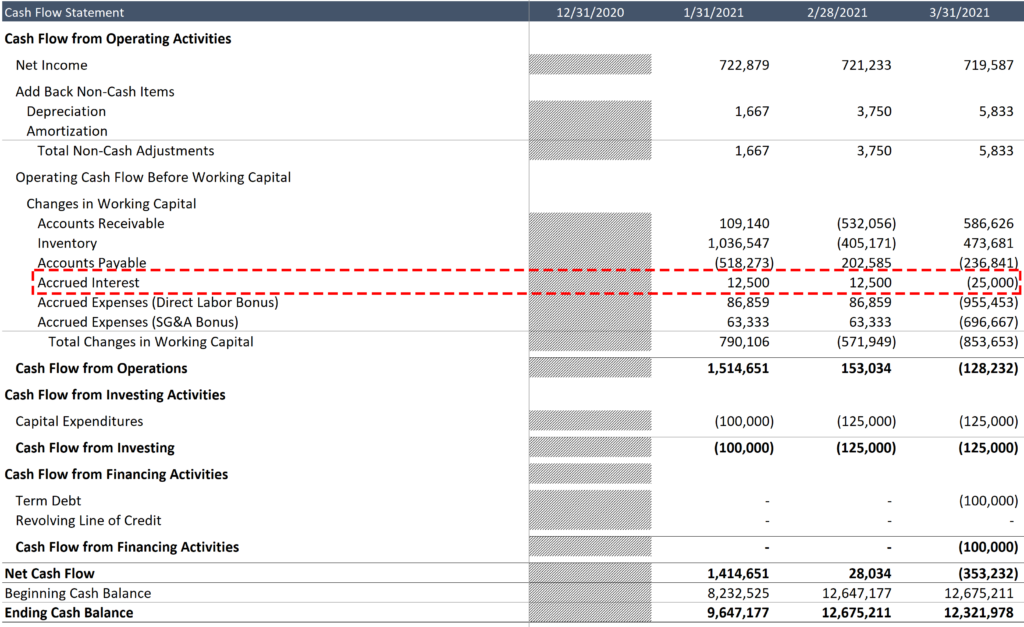You may notice that the cash outflow on the cash flow statement is equivalent to -\$25,000 in period 3/31/2021, which does not match interest paid of \$37,500 on the debt schedule. This is due to the fact that \$12,500 of interest expense is included in net income, the first line on the cash flow statement.

Note: FASB requires that this sum be included in cash flow from operations. It should never be included under cash flow from financing activities.